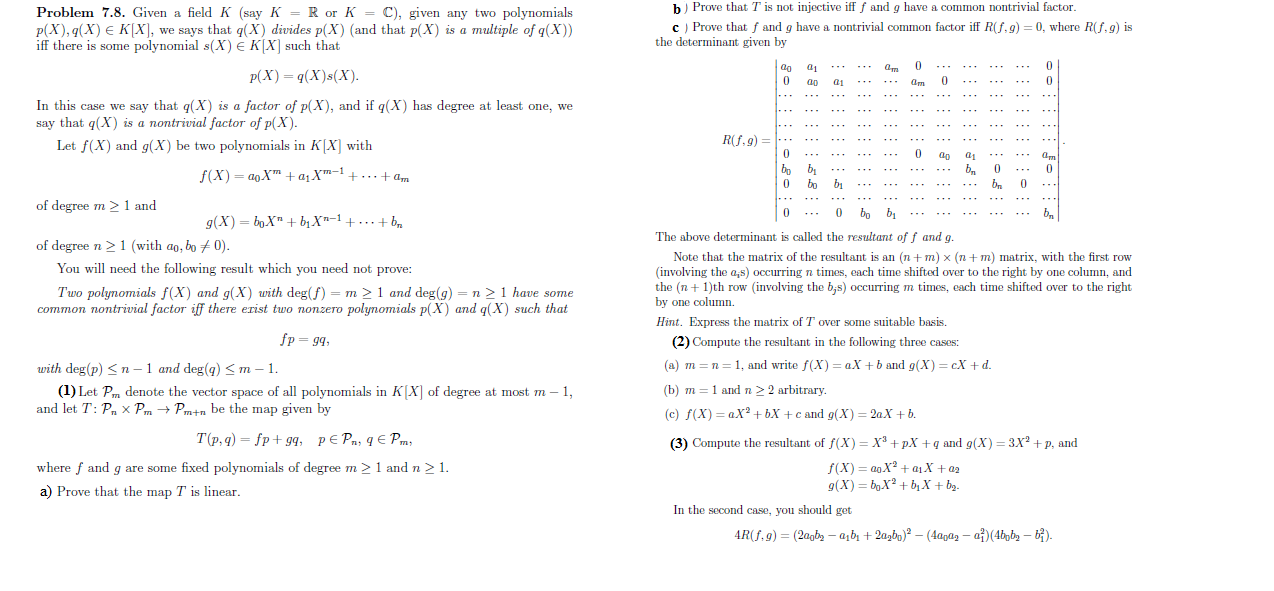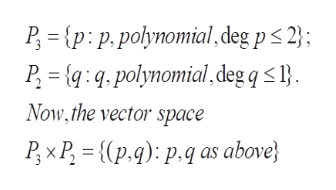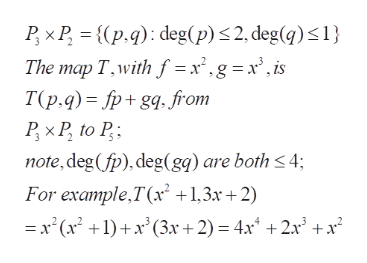# b Prove that T is not injective ifff and g have a common nontrivial factorProblem 7.8. Given a field K (say K = R or K = C), given any two polynomialsp(X), q(X) E K[X], we says that q(X) divides p(X) (and that p(X) is a multiple of q(X))iff there is somec Prove that f and g have a nontrivial common factor iff Rf,g) = 0, where R(f, g) isthe determinant given bypolynomial s(X) e K[X] such that0mP(X) q(X)s(X)000арamIn this case we say that q(X) is a factor of p(X), and if q(X) has degree at least one, wesay that q(X) is a nontrivial factor of p(X-.Rf.g)Let f(X) and g(X) be two polynomials in K[X] with-0apamb,00f(X) aoX a1X1...+a,.. .0bo...-of degree m1 andbo bb100g(X) boX bX-1+ ... + b.....The above determinant is called the resultant off and gof degree n 1 (with ao, bo 0).You will need the following result which you need not prove:Two polynomials f(X) and g(X) with deg(f) = m 2 1 and deg(g) = n 2 1 have somecommon nontrivial factor iff there erist two nonzero polynomials p(X) and q(X) such thatNote that the matrix of the resultant is an(n + m) x (n + m) matrix, with the first row(involving the a,s) occurring n times, each time shifted over to the right by one column, andthe (n1th row (involving the b,s) occurring m times, each time shifted over to the rightby one columnHint. Express the matrix of T over some suitable basis.fp g4(2) Compute the resultant in the following three cases(a) m n 1, and write f(X) aX +b and g(X) cX +dwith deg(p)n1 and deg(g) m1(b) m 1 and n 2 2 arbitrary(1) Let Pm denote the vector space of all polynomials in K[X] of degree at most m - 1,and let T: P x PmPmtn be the map given by>(c) f(X) aX2+bX +c and g(X) 2aX +b.Т(р,9) — fp+ g9, р€Pа, q€ Рm,(3) Compute the resultant of f(X)= X3 +pX q and g(X)= 3X2 p, andfixed polynomials of degree mwhere f and q are somef(X) aoX aj X +a2g(X) bXb X + b21 and n 2 1.a) Prove that the map T is linearIn the second case, you should get4R(f.9)= (2agb2 -a,b+2agbn) - (4agaz - ai)(4bpb2 - t).

Question
3 views

Please, help me with a step by step solutions to problem (Q1 a,b,c) and I will be very grateful to you. I don't have a strong background in algebra. I hope you will help.help_outlineImage Transcriptioncloseb Prove that T is not injective ifff and g have a common nontrivial factor Problem 7.8. Given a field K (say K = R or K = C), given any two polynomials p(X), q(X) E K[X], we says that q(X) divides p(X) (and that p(X) is a multiple of q(X)) iff there is some c Prove that f and g have a nontrivial common factor iff Rf,g) = 0, where R(f, g) is the determinant given by polynomial s(X) e K[X] such that 0 m P(X) q(X)s(X) 0 0 0 ар am In this case we say that q(X) is a factor of p(X), and if q(X) has degree at least one, we say that q(X) is a nontrivial factor of p(X - . Rf.g) Let f(X) and g(X) be two polynomials in K[X] with - 0 ap am b, 0 0 f(X) aoX a1X1...+a, .. . 0 bo ... - of degree m 1 and bo b b 10 0 g(X) boX bX-1+ ... + b .. ... The above determinant is called the resultant off and g of degree n 1 (with ao, bo 0). You will need the following result which you need not prove: Two polynomials f(X) and g(X) with deg(f) = m 2 1 and deg(g) = n 2 1 have some common nontrivial factor iff there erist two nonzero polynomials p(X) and q(X) such that Note that the matrix of the resultant is an (n + m) x (n + m) matrix, with the first row (involving the a,s) occurring n times, each time shifted over to the right by one column, and the (n1th row (involving the b,s) occurring m times, each time shifted over to the right by one column Hint. Express the matrix of T over some suitable basis. fp g4 (2) Compute the resultant in the following three cases (a) m n 1, and write f(X) aX +b and g(X) cX +d with deg(p)n1 and deg(g) m 1 (b) m 1 and n 2 2 arbitrary (1) Let Pm denote the vector space of all polynomials in K[X] of degree at most m - 1, and let T: P x Pm Pmtn be the map given by > (c) f(X) aX2+bX +c and g(X) 2aX +b. Т(р,9) — fp+ g9, р€Pа, q€ Рm, (3) Compute the resultant of f(X)= X3 +pX q and g(X)= 3X2 p, and fixed polynomials of degree m where f and q are some f(X) aoX aj X +a2 g(X) bXb X + b2 1 and n 2 1. a) Prove that the map T is linear In the second case, you should get 4R(f.9)= (2agb2 -a,b+2agbn) - (4agaz - ai)(4bpb2 - t). fullscreen
check_circle

Step 1

Problem concerns properties of linear maps and polynomials over fields

Step 2

(a) Consider a specific example of the problem with m=2 and n=3, f = x^2 (deg g =m=2), g(x) = x^3 (deg g=n=3)help_outlineImage TranscriptioncloseP p p.polynomial, deg p < 2} ; P , polynomial, deg q < l} Now,the vector space PxP {p.q) p.q as above} fullscreen
Step 3

The map T(p,q) explained...help_outlineImage TranscriptionclosePxPp.) deg(p)s2, deg(g)s1 The map T, with fx.g =x,is T(p.q) fp+ gq. from Р. x Р. 1о Р; note, deg fp), deg(gq) are both < 4; For example,T(x2 +1,3x + 2 x(x2 1)x'(3x+2) 4x +2x3 +x2 fullscreen

### Want to see the full answer?

See Solution

#### Want to see this answer and more?

Solutions are written by subject experts who are available 24/7. Questions are typically answered within 1 hour.*

See Solution
*Response times may vary by subject and question.
Tagged in

### Math